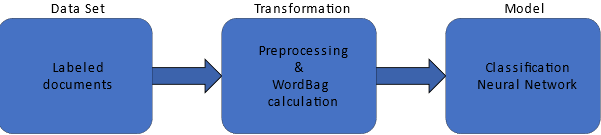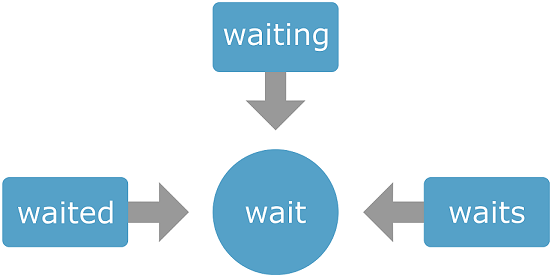Text classification is a machine learning technique that assigns predefined categories to open text.

Text classifiers can organize, structure, and categorize virtually any type of text, from documents, medical studies, and archives to the entire web. This post explains this Text Mining technique, its stages, and procedures. Neural Designer contains this machine learning technique so that you can apply it in practice. You can download a free trial here.

## Introduction

The categorization or classification of information is one of the most widely used branches of Text Mining. The premise of classification techniques is simple: starting from a set of data with an assigned category or label, the objective is to build a system to identify the existing documents’ patterns for determining their class. During the construction of the artificial intelligence model, the aim is to minimize the error between the categories predicted by the system and the real ones previously assigned.

Some examples of applications for text classification are spam detection, sentiment analysis, hate speech detection, and fake news detection.

Depending on the number of document classes, text classification problems can be:

1. Binary: here, the model must identify whether a document belongs to a class. An example of this problem is spam detection, where we must determine whether an email is spam.
2. Multiple: in this problem, each document must be assigned a class from a list of different ones. An example is sentiment analysis: emotions (happiness, sadness, joy,…) are the classes for this problem.

It is essential to highlight the difficulty of the data labeling process. Generally, experts in the field of application carry out this process manually.This image resumes the text classification training process.

We can divide the text classification process into the following steps:

1. Data processing and transformation
2. Model training
3. Testing analysis

## 1. Data processing and transformation

The transformation process in a classification problem comprises two stages: normalization and numerical representation.

### Normalization

Sometimes, in classification problems, the computational cost is very high, and reducing the number of input variables helps obtain better results faster. For this purpose, document normalization is generally applied. This process consists of using some of the following techniques for reducing the number of input words:

1. Lowercase transformation: for example, “LoWerCaSE” is transformed into “lowercase.”
2. Punctuation signs and special characters removal: punctuation signs and special characters like “;”,” #”, or “=” are removed.
3. Stop words elimination: Stop words are commonly used words in any language that don’t provide any information for our model. For example, some stop words in the English language are “myself”, “can”, and “under”.
4. Short and long word deletion: short words are eliminated because they do not provide much information, for example, the word “he”. On the other hand, long words are eliminated because of their low frequency in the documents.5. Stemming: Every word is composed of a root, a lemma (or lexeme), the part of the word that does not vary and indicates its central meaning, and a morpheme, particles that are added to the root to form new words.
The stemming technique replaces each word with its lemma to obtain fewer input words.

Once we have processed and normalized the documents, we must transform them into a numerical format that neural networks can process.

### Numerical representation

The intuition behind this idea is based on representing documents as vectors in an n-dimensional vector space. These vectors can be interpreted (and used) by the neural network to perform different tasks. One of the simplest traditional text representation techniques is Bag of Words.

### Bag of Words

Bag-of-Words (BoW) consists of constructing a dictionary for the working dataset and representing each document as a count of the words in it. In this type of representation, the document is represented by a vector length equal to the number of words in the dictionary. Each vector element is the number of times each token is used in the document.

water steak want don’t the some and I
“I want some water.” 1 0 1 0 0 1 0 1
“I want the steak.” 0 1 1 0 1 0 0 1
“I want steak, and I want water.” 1 1 1 0 0 0 1 1
“I don’t want water.” 1 0 1 1 0 0 0 1

We call this method “bag of words” since it does not represent the order of the words. It is also important to note that if new documents were introduced whose words are not present in the vocabulary, they could be transformed by omitting the unknown words.

The BoW model has several drawbacks in its use. One of the most relevant is that when the corpus size is considerable, the vocabulary size is consequently increased. Therefore, very sparse vector sets are obtained, with many zeros and large sizes, which implies a higher memory consumption.

## 2. Model training

Once the document’s numerical representation has been obtained, we can start model training using a classification neural network. A classification neural network usually requires a scaling layer, one or several perceptron layers, and a probabilistic layer.

## 3. Testing analysis

As with any classification problem, model evaluation is essential. However, in text classification problems, evaluation measures are not absolute, as they depend on the specific classification task: classifying medical texts is not the same as classifying whether a review is positive or negative. Therefore, the most usual thing to do is to look in the literature for baselines for similar tasks and compare with them to see if we are getting acceptable results.

### Confusion matrix

In the confusion matrix, the rows represent the target classes in the data set, and the columns represent the predicted output classes from the neural network.

The following table represents the confusion matrix:

Predit class 1 Predit class N
Red class 1 # # #
# # #
Red class N # # #

### Accuracy

The proportion of correctly classified documents from the total for which the model predicted the class c.
div style=”max-width: 100%; overflow-x:auto”>
$$precision = frac{# true positives}{# true positives + # false positives}$$

### Recall

The proportion of correctly classified documents among all documents in training set with class c.
$$recall = frac{# true positives}{# true positives + # false negatives}$$

### F1-Score

Generally, a good classifier should balance accuracy and recall. For this purpose, we use the F1-score metric, which considers both parameters. This score penalizes the total value if either is too low.
$$F1 = frac{2·precision·recall}{precision + recal}$$

In addition, we must move between the line of overfitting and underfitting to arrive at a quality classifier.
An under-fitted model has low variance, which means that whenever the same data is introduced, the same prediction is obtained, but its prediction is too far from reality. This phenomenon occurs when the model has insufficient training data to find the existing patterns in the data.

On the other hand, a way to achieve the optimal working point is to evaluate the model with data that has not been trained and has never been seen. For this reason, it is advisable to subdivide the corpus into multiple subsets (training, testing, and selection).

## Conclusions

Text classification is one of the most widely used Text Mining techniques today, with applications ranging from classifying reviews into positive and negative to sorting support messages by urgency.

This article has seen the different stages of text classification problems: data processing and transformation, model training, and testing analysis. Following these steps, we can build the best text classification models.